# Barrels

Peter has 42 barrels. One-sixth of them are filled with lemonade, one third is filled with wine and half is empty. Calculate how many barrels are with each filling.

Correct result:

a =  7
b =  14
c =  21

#### Solution: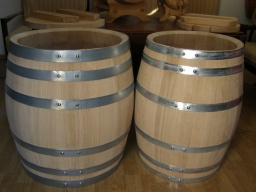We would be pleased if you find an error in the word problem, spelling mistakes, or inaccuracies and send it to us. Thank you!Tips to related online calculators
Need help calculate sum, simplify or multiply fractions? Try our fraction calculator.

## Next similar math problems:

• One third 2One third of all students in class live in a house. If here are 42 students in a class, how many of them live in house?
• One sixth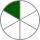How many sixths are two thirds?
• The King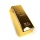The King wants to divide his sons equally. He has 42 rubies and 45 diamonds. How many sons and how will they share them?
• Empty and fullAn empty can has a mass of 1/6 lb. When it is filled with sand, it has a mass of 7/12 lb. Find the mass of the sand in the can?
• Caleb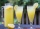Caleb is making lemonade for a party. He has 5 gallons of lemonade. He is putting 1/3 cubic inch of lemonade in a cup for each guest. How many guests are going to be at the party?
• Playing Cards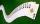Kara has 2 times more cards than Dana, Dana has 4× less than Mary. Together they have 728 cards. How many cards has each of them?
• Bar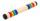Half of the bar is green, the third is yellow and the remaining 40cm is red. How long is a bar?
• Candies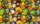There are 150 candies in the bowl. Ivan took 7 thirtieths, Charles 6 fifteenths, and Thomas 3 tenths. How many sweets are left in the bowl?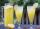How many 1/4 cup servings are in 2 and 1/3 cups of lemonade?
• Factors 2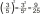A number has 3 identical factors. If 1 of them is 18. Find the number step by step
• Gasoline price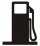At 34.4 cents per liter, how much will 42 liters of gasoline cost?
• Report 2A School reports students to teacher ratio of 6:1. If there are 45 teachers in the School, how many students are there?
• Five girls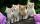Five girls traveling by bus. Each holds in each hand two baskets. In each basket are four cats. Each cat has three kittens. Two girls exits bus. How many legs are in the bus?
• Number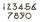What number I think? The third is 6 and half is 2 more than the its quarter.
• Moneys in triple ratioMilan, John and Lili have a total 344 euros. Their amounts are in the ratio 1:2:5. Determine how much each of them has?
• Fruits 2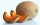An apple costs 7p and a melon costs seven times more. How much will 6 apples and 2 melons cost
• How manyHow many numbers are less than 222 with a digit sum is 8?